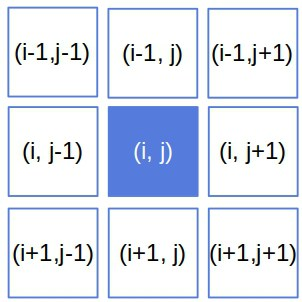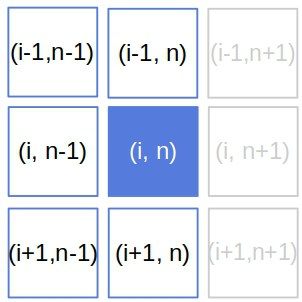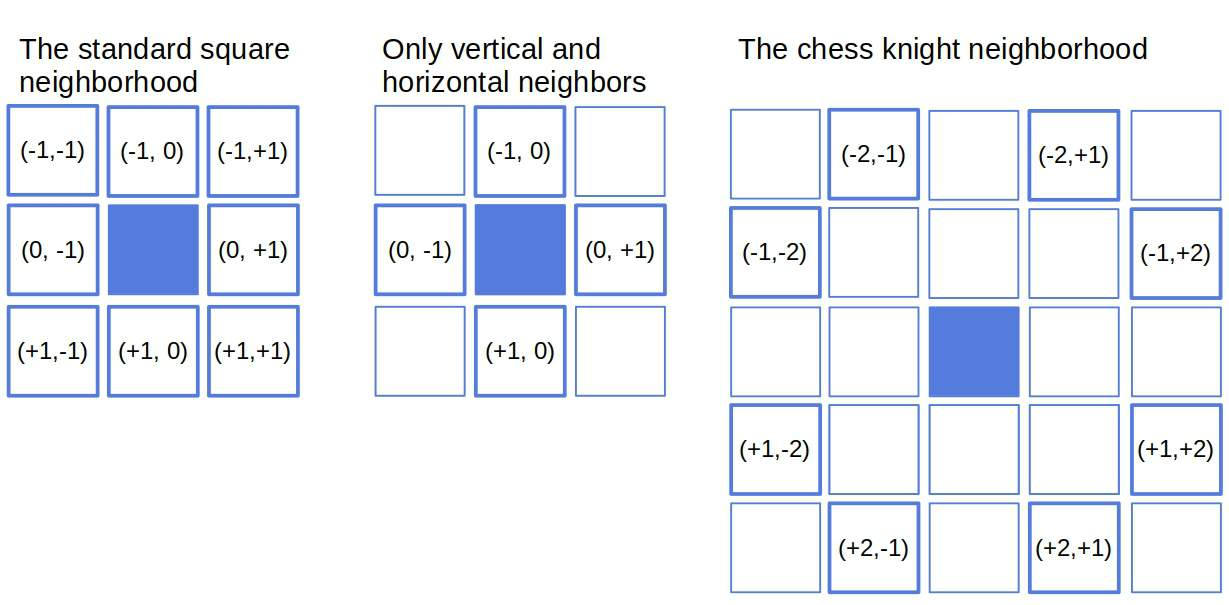## 1. Introduction

In this tutorial, we’ll show how to find neighbors of a cell in a two-dimensional matrix.

## 2. Neighbors

Let’s say we have anmatrix. We want to get all the neighbors of, the cell in the-th row and-th column ().

Usually, we define a cell’s neighbors as all the cells it borders with horizontally, vertically, or diagonally:The vertical neighbors ofareand. They’re in the same column as, right above and below it. Similarly, its horizontal neighbors areand: the cells right to its left and right.

The diagonal neighbors are in the corners of the neighborhood square. The top left is, the top right, the bottom left is, and the bottom right.

## 3. Iterating Over Neighbors

From the above, it follows that we can iterate over all the combinations ofandto get the neighbors of. In doing so, we need to take care of two things.

First, it makes no sense to returnas its own neighbor, so we’ll omit the combination. Additionally, ifis at a border, some combinations will be illegal since they’ll be outside the matrix, so we need to skip them. For example, if, there are no neighbors to the right of:Taking those two points into account, we get the neighbors ofas follows:A problem with this approach is that the neighborhood is hard-coded. So, if we want to define neighbors differently, we need to change the loops. Depending on the neighborhood definition, that can be cumbersome and prone to errors.

## 4. Iterating Over Offsets

A more flexible approach is to iterate over offsets. For instance, the offset oftois. So, if we have the offsets, we can iterate over them to get the neighbors of:We can define a neighborhood in various ways:Although more flexible, this approach requires us to generate the offsets first. One way to do so is to use the corresponding distance function. For example, the Manhattan distances in the standard neighborhood are either 1 or 2. So, the offsets are in the set:We can also plug in the boundary checks so that all the offsets are safe:In that case, we don’t need any checks in the above algorithm.

## 5. Conclusion

In this article, we showed how to find neighbors of a cell in a 2D matrix.